2. Limits

# Chapter 2 Review Exercises

True or False. In the following exercises, justify your answer with a proof or a counterexample.

1. A function has to be continuous at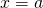if the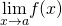exists.

2. You can use the quotient rule to evaluate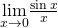.

#### Solution

False

3. If there is a vertical asymptote atfor the function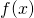, then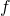is undefined at the point.

4. Ifdoes not exist, thenis undefined at the point.

#### Solution

False. A removable discontinuity is possible.

5. Using the graph, find each limit or explain why the limit does not exist.

1.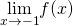2.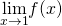3.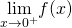4.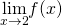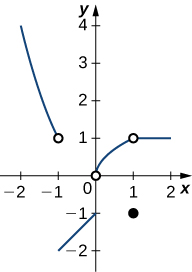1, starting at the open circle at (1,1).”>

In the following exercises, evaluate the limit algebraically or explain why the limit does not exist.

6.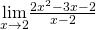#### Solution

5

7.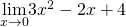8.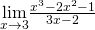#### Solution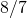9.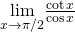10.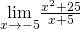#### Solution

DNE

11.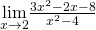12.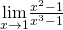#### Solution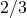13.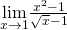14.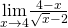#### Solution

−4

15.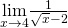In the following exercises, use the squeeze theorem to prove the limit.

16.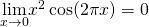#### Solution

Since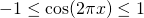, then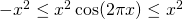. Since, it follows that.

17.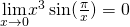18. Determine the domain such that the function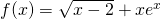is continuous over its domain.

#### Solution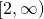In the following exercises, determine the value of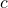such that the function remains continuous. Draw your resulting function to ensure it is continuous.

19.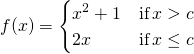20.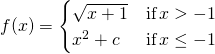#### Solution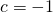In the following exercises, use the precise definition of limit to prove the limit.

21.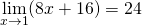22.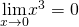#### Solution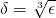23. A ball is thrown into the air and the vertical position is given by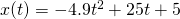. Use the Intermediate Value Theorem to show that the ball must land on the ground sometime between 5 sec and 6 sec after the throw.

24. A particle moving along a line has a displacement according to the function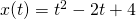, where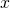is measured in meters andis measured in seconds. Find the average velocity over the time period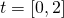.

#### Solution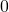m/sec

25. From the previous exercises, estimate the instantaneous velocity at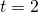by checking the average velocity within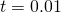sec.